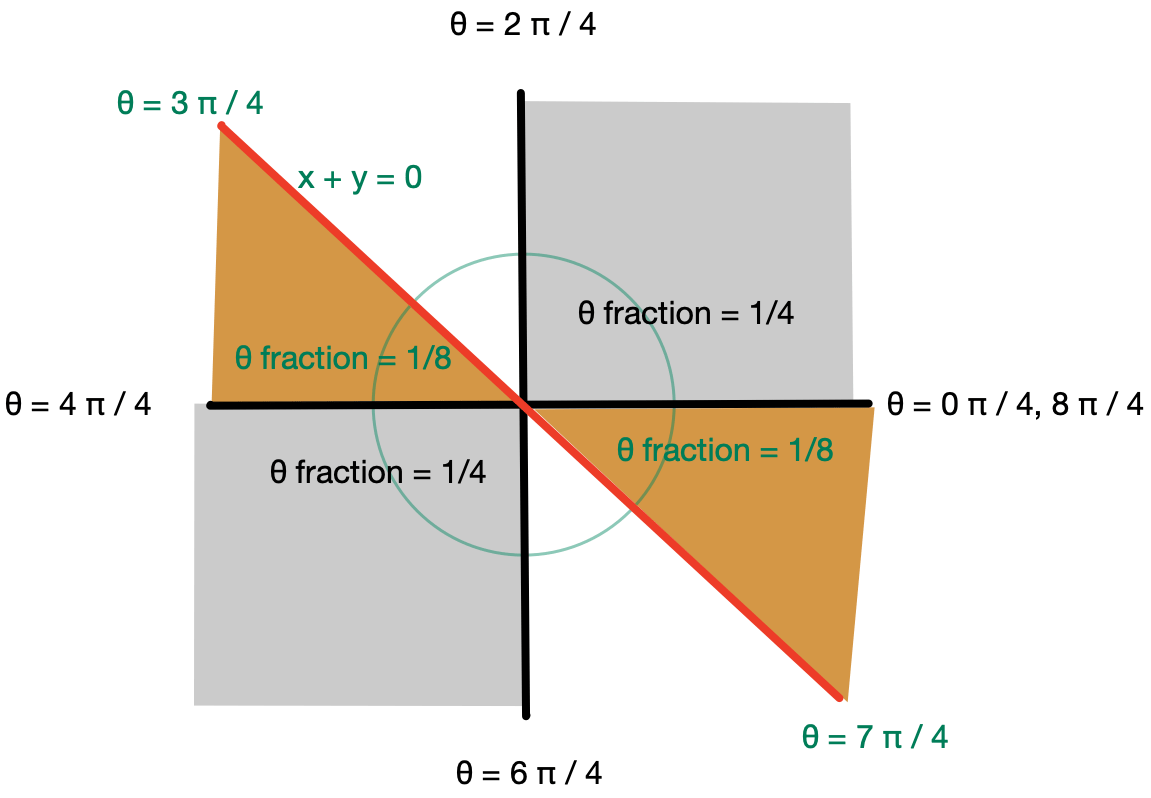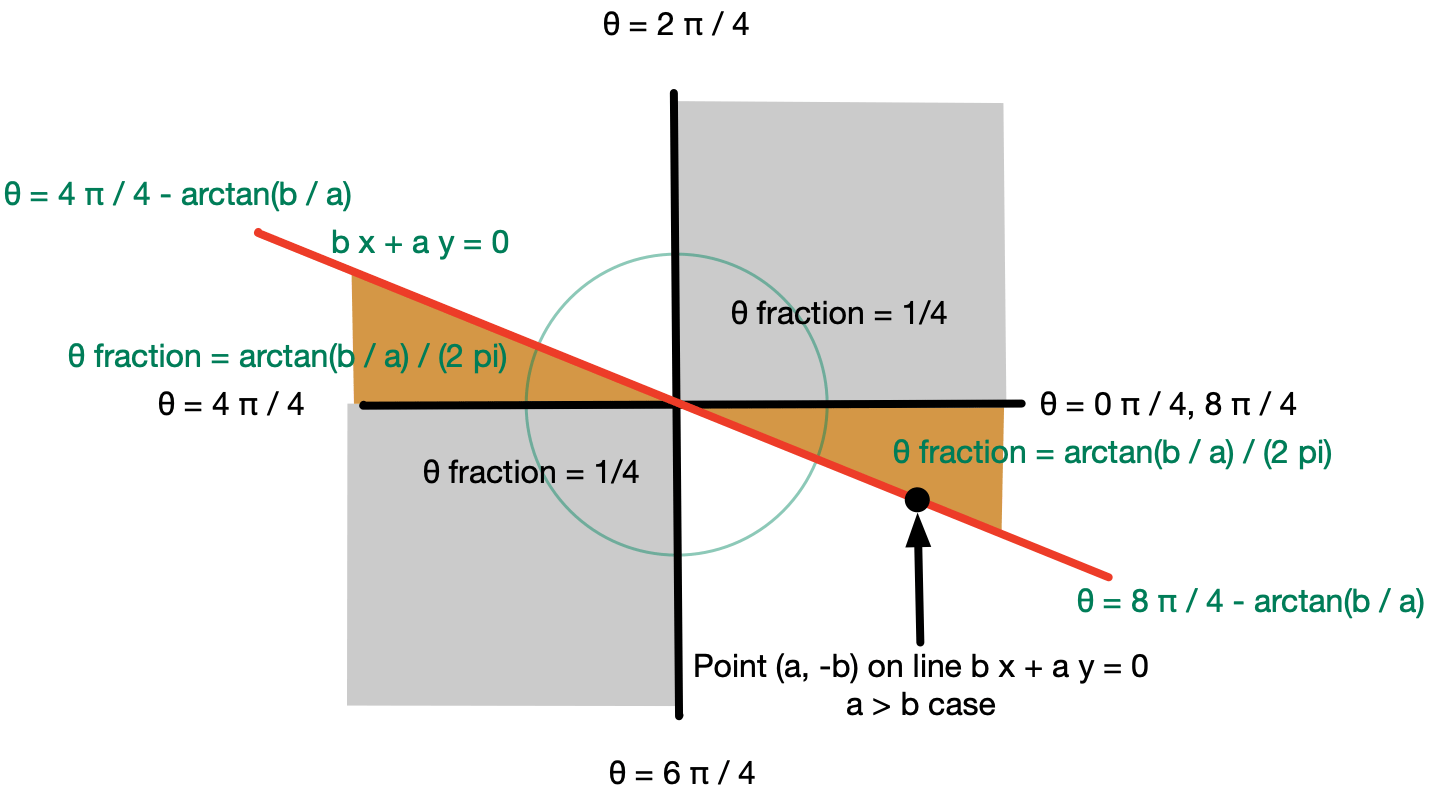# Tilting at Sign

Establishing the “L1L2 AUC” equals `1/2 + arctan(1/sqrt(π - 3)) / π` (`≅ 0.8854404657887897`) used a few nifty lemmas. One of which I am calling “the sign tilting lemma.”The sign tilting lemma is:

For X, Y independent mean zero normal random variables with known variances `sx2` and `sy2`, what is `P[(X + Y ≥ 0) = (X ≥ 0)]`?

It turns out it is: `1/2 + arctan(sx / sy) / π`.

This is solving: how much does knowing a fraction of the variance tell you about the sign of a sum?

Let’s check if this is right in a few cases.

• For `sx / sy` large we expect `P[(X + Y ≥ 0) = (X ≥ 0)]` near 1 (`X` dominates `X + Y`, making `X` and `X + Y` highly correlated). Our formula agrees with this.
• For `sx / sy` near zero we expect `P[(X + Y ≥ 0) = (X ≥ 0)]` near 1/2 (`Y` dominates `X + Y`, making `X` and `X + Y` nearly independent). Our formula agrees with this.
• For `sx / sy = 1` we expect `P[(X + Y ≥ 0) = (X ≥ 0)] = 3/4`. Our formula agrees with this.

The reason we have that `sx / sy = 1` implies `P[(X + Y ≥ 0) = (X ≥ 0)] = 3/4` is as follows. We only get inequality when `X` and `Y` have different signs (chance 1/2 of that by symmetry of `X` to `Y`) and `|Y| > |X|` (an independent chance 1/2 of that, again by a symmetry argument). So the mismatch chance is 1/4, meaning the match chance is 3/4. In this case the following diagram shows that 3/4 of the angles between `(0, 0)` and `(x, y)` are in the shaded regions where `sign(x) = sign(x + y)`And this completes the `sx / sy = 1` case, as in this case `(x, y)` is spherically symmetric (generates all angles uniformly at random).

For the general case we convert the question of if `sx u ≥ sy v` (for `u`, `v` independent normal mean zero variance 1 random variables) to checking if the point `(sx u, sy v)` is above or below the line `x + y = 0`. This in turn is equivalent to checking if the point `(u, v)` is above or below the line `sy x + sx y = 0` (notice the swap of coefficients, as this is the algebra for checking if a point is above or below a line!). This swap is a bit tricky to visualize, it is a bit of how geometry and algebra differ in viewpoint.

The advantage of the last check being: we are again in spherically symmetric situation with all angles generated uniformly. We can illustrate the geometry as follows. We put on the x-axis `u = X / sx`, and on the y-axis `v = Y / sy` to get the uniform distribution on angles. In this notation we use `a = 1 / sx` and `b = 1 / sy`. This can be very confusing, as we get one reversal from how we check above/below lines and another (cancelling reversal) by inverting `(u, v) → (X, Y)` to `(X, Y) → (u, v)`. In honest practice, we use the diagram to work out the answer has to have `arctan(sx / sy)` or `arctan(sy / sx)` in it, and pick the one that works (though in math one has to pretend to never have such difficulty or to use a dirty move to get out of it!).Adding up the indicated areas of the shaded regions completes our argument for the lemma.

To conclude, we can rephrase or lemma as follow.

If a mean zero normal random variable determines a `f` fraction of the variance of a mean zero sum of independent normal random variables, then its sign matches the sign of the sum a `1/2 + arctan(sqrt(f / (1-f))) / π` fraction of the time. That is: it gives a `arctan(sqrt(f / (1-f))) / π` advantage in guessing the sign of the sum. For small `f` this advantage is approximately `sqrt(f) / π`.

Categories: Mathematics

Tagged as: